Note: The other languages of the website are Google-translated. Back to English

## How to change or convert number to text in Excel?

Sometimes you may not want the numbers in the cells getting involved in calculating, or you may want to display leading zeros in numbers in cells. For doing so, you may need to change a number into text. The following methods can convert numbers in cells to text in Excel.

Convert number to text with Text function

Convert number to text with Format Cells command

#### Convert number to text with Text function

If you are familiar with Microsoft Excel's formulas, you can convert numbers in cells to text with Text function.

If you just only want to convert the number to text without any formatting, you can use the formula: =TEXT(A1,"0");

1. In cell E1, please enter the formula =TEXT(A1,"0").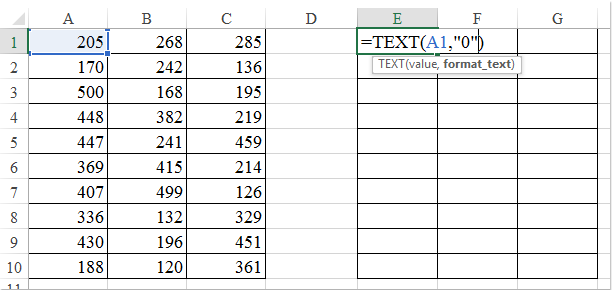2. Then press Enter key. And select the cell E1, drag the fill handle over the range of cells that you want to apply this formula.See screenshot: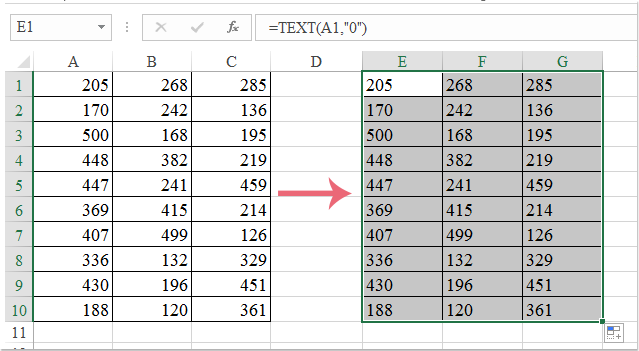Note: As the data is formula, you can change the formulas to values. Copy the cells and right-click where you want to paste the values and select Paste special > Values.

If you want to display the leading zero in numbers, you can use this formula: =TEXT(A1,"00000"); such as convert 23 to 00023.

As the above steps, if you apply this formula, you will get the following result:And if you want to convert the numbers to text which keep several decimal digits, you can use this formula: =TEXT(A1,"0.000"). Such as convert 23 to 23.000.

Also with above steps, if you apply this formula, you will get the result as the following screenshots: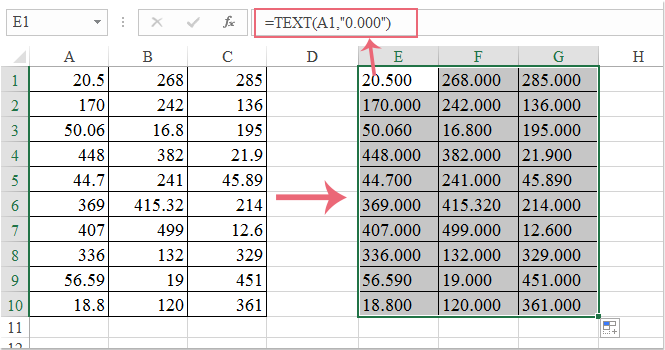Note: you can define the text style. For example, if you want to convert the numbers to text with a telephone number format, you can replace the "000" with "00-00000", or others.

 Convert or spell out numbers to English or Chinese currency: With Kutools for Excel's Numbers to Words feature, you can spell out numbers into English and Chinese words in Excel.For example, it can spell out the "12.75" to "Twelve dollars and seventy-five cents".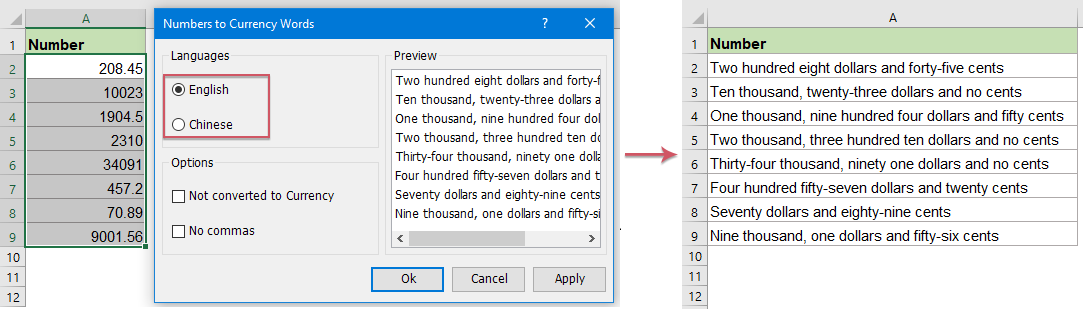Kutools for Excel: with more than 300 handy Excel add-ins, free to try with no limitation in 30 days.

#### Convert number to text with Format Cells command

Microsoft Excel's Format Cells command is also able to convert numbers to text.

1. Select the numbers that you want to convert to text.

2. Right click the selected range, and choose the Format Cells item from context menu. See screenshot: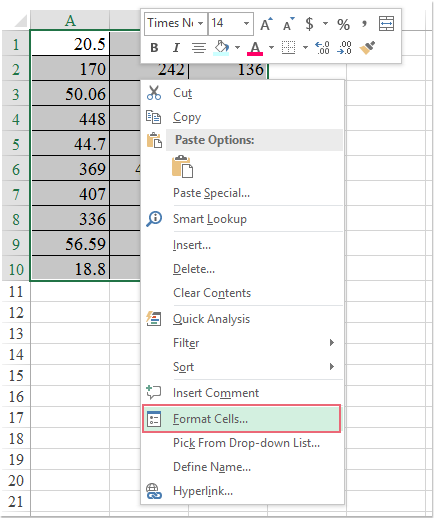3. In the Format Cells dialog box, select the Text item in the Category box under Number tab, and then click the OK button. See screenshot:4. Then numbers in selected range are converted into text.

#### Convert number to text with Kutools for Excel

If you have Kutools for Excel installed, it will be easier for you to convert numbers in cells to text with Convert between Text and Number.

 : with more than 300 handy Excel add-ins, free to try with no limitation in 30 days.

After installing Kutools for Excel, please do as below:

1. Select the range with numbers that you want to change to text.

2. Click Kutools > Content > Convert between Text and Number. See screenshot: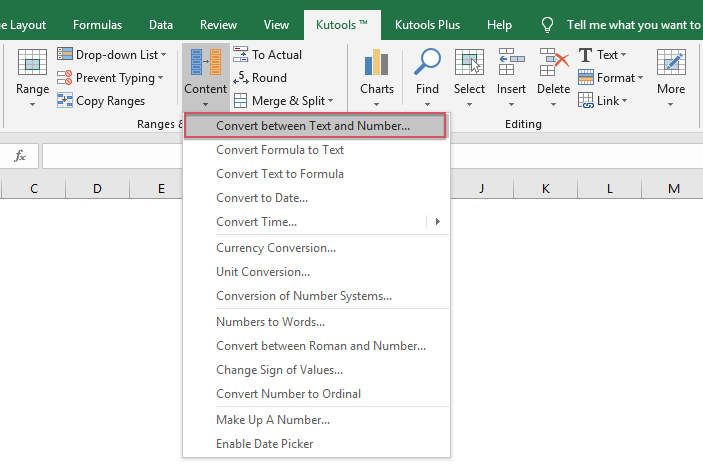3. In the Convert between Text and Number dialog box, check the Number to text option, and then click the OK or Apply button. And all numbers have been converted to text in the original range. See screenshot:Kutools for Excel: with more than 300 handy Excel add-ins, free to try with no limitation in 30 days.

Related article:

### The Best Office Productivity Tools

#### Kutools for Excel Solves Most of Your Problems, and Increases Your Productivity by 80%

• Reuse: Quickly insert complex formulas, charts and anything that you have used before; Encrypt Cells with password; Create Mailing List and send emails...
• Super Formula Bar (easily edit multiple lines of text and formula); Reading Layout (easily read and edit large numbers of cells); Paste to Filtered Range...
• Merge Cells/Rows/Columns without losing Data; Split Cells Content; Combine Duplicate Rows/Columns... Prevent Duplicate Cells; Compare Ranges...
• Select Duplicate or Unique Rows; Select Blank Rows (all cells are empty); Super Find and Fuzzy Find in Many Workbooks; Random Select...
• Exact Copy Multiple Cells without changing formula reference; Auto Create References to Multiple Sheets; Insert Bullets, Check Boxes and more...
• Extract Text, Add Text, Remove by Position, Remove Space; Create and Print Paging Subtotals; Convert Between Cells Content and Comments...
• Super Filter (save and apply filter schemes to other sheets); Advanced Sort by month/week/day, frequency and more; Special Filter by bold, italic...
• Combine Workbooks and WorkSheets; Merge Tables based on key columns; Split Data into Multiple Sheets; Batch Convert xls, xlsx and PDF...
• More than 300 powerful features. Supports Office / Excel 2007-2021 and 365. Supports all languages. Easy deploying in your enterprise or organization. Full features 30-day free trial. 60-day money back guarantee.#### Office Tab Brings Tabbed interface to Office, and Make Your Work Much Easier

• Enable tabbed editing and reading in Word, Excel, PowerPoint, Publisher, Access, Visio and Project.
• Open and create multiple documents in new tabs of the same window, rather than in new windows.
• Increases your productivity by 50%, and reduces hundreds of mouse clicks for you every day!No ratings yet. Be the first to rate!
This comment was minimized by the moderator on the site
i need changes number to text ex: 2 -> two can u give the answer pls tx alot
This comment was minimized by the moderator on the site
[quote]i need changes number to text ex: 2 -> two can u give the answer pls tx alotBy indah[/quote] I also want to know the answer. Thanks
This comment was minimized by the moderator on the site
First you need to ascertain that upto how many digits you are going to use as the highest value. For Example it is 5 digits. In the cell type any 5 digit number for example 59427. Now in a different cell convert this into decimal places of 5 digits i.e. 59427/10000 = 0.59427 Now you can use the function "MID" to break this into 5 different digits i.e. 5, 9, 4, 2, 7 By using the if formula convert these into words like =IF(A3=5,"Fifty","") and finally use the function "CONCATENATE" to bring these together. This is the only way possible. If you need I can send you the sample excel sheet. Regards
This comment was minimized by the moderator on the site
Same Question Man! Axit Mehta
This comment was minimized by the moderator on the site
First you need to ascertain that upto how many digits you are going to use as the highest value. For Example it is 5 digits. In the cell type any 5 digit number for example 59427. Now in a different cell convert this into decimal places of 5 digits i.e. 59427/10000 = 0.59427 Now you can use the function "MID" to break this into 5 different digits i.e. 5, 9, 4, 2, 7 By using the if formula convert these into words like =IF(A3=5,"Fifty","") and finally use the function "CONCATENATE" to bring these together. This is the only way possible. If you need I can send you the sample excel sheet. Regards
This comment was minimized by the moderator on the site
HELLO i WANT TO CONVERT NUMBER INTO TEXT.. FOR Eg 0-SERVICE MODE 1-BACKWASH MODE 2-CIP MODE 3-MANTAINENCE MODE 4-NO MODE MY DATA COMES FROM PLC PROGRAMMING TO EXCEL THROUGH VB SCRIPT HOW CAN I DO IT?
This comment was minimized by the moderator on the site
Use the "IF" function.
This comment was minimized by the moderator on the site
I want to convert number into text. for ex. 123- One hundered and twenty three. in this way. Is there any comand for changing so.
This comment was minimized by the moderator on the site
I also want to convert like that if you got the solution please tell me also. :-)
This comment was minimized by the moderator on the site
dear sir i want typing the number in excel then next convert to words sample-1000-One thousand only
This comment was minimized by the moderator on the site
dear sir i want typing the number in excel then next convert to words sample-1000-One thousand only[/quote]
This comment was minimized by the moderator on the site
This comment was minimized by the moderator on the site
First you need to ascertain that upto how many digits you are going to use as the highest value. For Example it is 5 digits. In the cell type any 5 digit number for example 59427. Now in a different cell convert this into decimal places of 5 digits i.e. 59427/10000 = 0.59427 Now you can use the function "MID" to break this into 5 different digits i.e. 5, 9, 4, 2, 7 By using the if formula convert these into words like =IF(A3=5,"Fifty","") and finally use the function "CONCATENATE" to bring these together. This is the only way possible. If you need I can send you the sample excel sheet. Regards
This comment was minimized by the moderator on the site
Would appreciate a sample please :) Thanks!
This comment was minimized by the moderator on the site
would you please explain the how to remove to from currency name from formula
This comment was minimized by the moderator on the site
Hi, I want to convert figure in text, i.e. 102 means one zero two or one hundred two. etc.
This comment was minimized by the moderator on the site
I need to change a number to text eg. in a cell I enter 202, I need to display this number in another cell with words eg. Two (hundred and two) Best Regards Yanek
This comment was minimized by the moderator on the site
I need to display a number of a cell in text format in another cell eg. A1=300 to be displayed at: J10 as text (Three hundred). Thanks
This comment was minimized by the moderator on the site
I want to convert number into text. for ex. 123- One hundered and twenty three. in this way. Is there any comand for changing so.
This comment was minimized by the moderator on the site
try using formula =rswords(123)
This comment was minimized by the moderator on the site
[quote]Hi, I want to convert figure in text, i.e. 102 means one zero two or one hundred two. etc.By SUDIPTA GHOSH[/quote] I am also want This ans If you get the ans please reply me.
This comment was minimized by the moderator on the site
Hi, I want to convert figure in text, i.e. 102 means one zero two or one hundred two. etc.
By Ripon

I am also want This ans If you get the ans please reply me.
By SUDIPTA GHOSH

I would like to convert number to ward text in indian rupees format.
e.g.-2500
In Word: Rupees Two Thousand Five Hundred Only.

By Ajay Ramteke
This comment was minimized by the moderator on the site
I want to convert text to number in excel sheet
This comment was minimized by the moderator on the site
Please see office help if covert number in text format.Thanks
This comment was minimized by the moderator on the site
I would like to convert number to ward text in indian rupees format. e.g.-2500 In Word: Rupees Two Thousand Five Hundred Only. If possible please help me.
This comment was minimized by the moderator on the site
convert number to ward text in indian rupees format.
This comment was minimized by the moderator on the site
Download Sivaword.xla Go to add-inn & add this in this function. after that click/select a cell & Write "=sivaword(A1) where A1 is a cell where You wrote your value which you want to convert in words in INR Currency. Thanks
This comment was minimized by the moderator on the site
Where we can down load. It is possible i need in USD
This comment was minimized by the moderator on the site
First you need to ascertain that upto how many digits you are going to use as the highest value. For Example it is 5 digits. In the cell type any 5 digit number for example 59427. Now in a different cell convert this into decimal places of 5 digits i.e. 59427/10000 = 0.59427 Now you can use the function "MID" to break this into 5 different digits i.e. 5, 9, 4, 2, 7 By using the if formula convert these into words like =IF(A3=5,"Fifty","") and finally use the function "CONCATENATE" to bring these together. This is the only way possible. If you need I can send you the sample excel sheet. Regards
This comment was minimized by the moderator on the site

123oldmonks1@gmail.com
This comment was minimized by the moderator on the site
guys....you need to make your own formula for that kind of conversion and finaly i make my own converter from numbers to words ex. SAR. 123 = One Hundred Twenty Three SAR.
This comment was minimized by the moderator on the site
Dear Provider, I need to convert number to text like as below e.g. 344835.10 = THREE HUNDRED FORTY FOUR THOUSAND EIGHT HUNDRED THIRTY FIVE & TEN CENTS ONLY. if you can help us it's better for my commercial work.
This comment was minimized by the moderator on the site
if i type 1 i want do display one in any other cell
This comment was minimized by the moderator on the site
thanks for great full help... pls do more for Spared Sheet Thank You
This comment was minimized by the moderator on the site
I wanted to have the formula to convert the numbers into text. e.g i write 105 in cell A1 and wanted to see (One hundred five riyals)in Cell B1. Thanks Iqbal
This comment was minimized by the moderator on the site
Dear Sir I want to change number into words eg: 1000 in words one thousand only
This comment was minimized by the moderator on the site
can i convert a numeric value into kannada words in M S Excel
This comment was minimized by the moderator on the site
change number into figure in Excel like 2 = Two Step1: press alt+F11 Step2: Insert à Module Step3: type ='wordnum(12)' will show `Twelve'. Option Explicit Public Numbers As Variant, Tens As Variant Sub SetNums() Numbers = Array("", "One", "Two", "Three", "Four", "Five", "Six", "Seven", "Eight", "Nine", "Ten", "Eleven", "Twelve", "Thirteen", "Fourteen", "Fifteen", "Sixteen", "Seventeen", "Eighteen", "Nineteen") Tens = Array("", "", "Twenty", "Thirty", "Forty", "Fifty", "Sixty", "Seventy", "Eighty", "Ninety") End Sub Function WordNum(MyNumber As Double) As String Dim DecimalPosition As Integer, ValNo As Variant, StrNo As String Dim NumStr As String, n As Integer, Temp1 As String, Temp2 As String ' This macro was written by Chris Mead - http://www.MeadInKent.co.uk If Abs(MyNumber) > 999999999 Then WordNum = "Value too large" Exit Function End If SetNums ' String representation of amount (excl decimals) NumStr = Right("000000000" & Trim(Str(Int(Abs(MyNumber)))), 9) ValNo = Array(0, Val(Mid(NumStr, 1, 3)), Val(Mid(NumStr, 4, 3)), Val(Mid(NumStr, 7, 3))) For n = 3 To 1 Step -1 'analyse the absolute number as 3 sets of 3 digits StrNo = Format(ValNo(n), "000") If ValNo(n) > 0 Then Temp1 = GetTens(Val(Right(StrNo, 2))) If Left(StrNo, 1) "0" Then Temp2 = Numbers(Val(Left(StrNo, 1))) & " hundred" If Temp1 "" Then Temp2 = Temp2 & " and " Else Temp2 = "" End If If n = 3 Then If Temp2 = "" And ValNo(1) + ValNo(2) > 0 Then Temp2 = "and " WordNum = Trim(Temp2 & Temp1) End If
This comment was minimized by the moderator on the site
There seems to be some errors in the above code - doesn't work quite right (for example, result when tested with "1" is "hundred and".
This comment was minimized by the moderator on the site
This is for All! Any body wishing to get your numbers to text (like in words) I have already created a VB some 12 years ago which used to work perfectly as I was doing some Financial projects that period of time....
Any body interested to transform the code to your excel can send me a mail on R-aviang@live.com. I will share the code. Thanks
Ravi Shankar S
This comment was minimized by the moderator on the site
this part here is not okay: If Left(StrNo, 1) "0" Then Temp2 = Numbers(Val(Lef t(StrNo, 1))) & " hundred" If Temp1 "" Then Temp2 = Temp2 & " and " how to correct that please? thanks
This comment was minimized by the moderator on the site
Dear Provider, I need to convert number to text like as below e.g. 344835.10 = THREE HUNDRED FORTY FOUR THOUSAND EIGHT HUNDRED THIRTY FIVE & TEN PAISA ONLY. if you can help us it's better for my commercial work.
There are no comments posted here yet